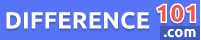Rational Number

A ratio can be defined as a comparison of quantities and is expressed in fraction form. A rational number can be expressed through fractions. For instance, in the fraction p/q, “p” is a numerator while “q” is a denominator. Both are integers. Here, a numerical denominator is natural (non-zero). All integers, fractions (which includes mixed ones), and decimals (including recurring and finite) are rational numbers